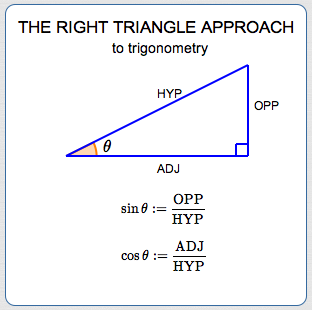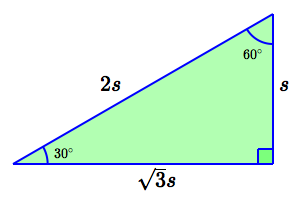﻿ the Right Triangle Approach to Trigonometry

# THE RIGHT TRIANGLE APPROACH TO TRIGONOMETRY

by Dr. Carol JVF Burns (website creator)
Follow along with the highlighted text while you listen!
• PRACTICE (online exercises and printable worksheets)

There are two basic approaches to trigonometry:

Both approaches were introduced in Introduction to Trigonometry.

Be sure to read this prior section, since it covers important notation and conventions.

## The Right Triangle Approach to Trigonometry

 Start with a right triangle (i.e., a triangle with a $\,90^{\circ}$ angle). (Don't despair! From these humble beginnings, tools for any triangle will be developed!) Focus attention on one of the two acute angles. Whichever one you choose, call it $\,\theta\,.$ Relative to $\,\theta\,,$ names are given to the lengths of the sides (see diagram at right): the side opposite (across from) $\,\theta\,$ is called OPP (for OPPosite) the non-hypotenuse side adjacent to (next to) $\,\theta\,$ is called ADJ (for ADJacent) The hypotenuse is the longest side in the triangle. It is the side opposite the right angle. The hypotenuse is called HYP (for HYPotenuse). Define: $$\begin{gather} \cssId{s19}{\sin\theta := \frac{\text{OPP}}{\text{HYP}}}\cr\cr \cssId{s20}{\cos\theta := \frac{\text{ADJ}}{\text{HYP}}}\cr\cr \end{gather}$$ (Recall that ‘$\,:=\,$’ means ‘equals, by definition’.) Since the hypotenuse is the longest side, both of these ratios are less than $\,1\,.$ There are four more trigonometric functions, each of which are defined in terms of sine and cosine. One of these is the tangent function: $$\cssId{s25}{\tan\theta \ :=\ \frac{\sin\theta}{\cos\theta}} \cssId{s26}{\ =\ \frac{\text{OPP}/\text{HYP}}{\text{ADJ}/\text{HYP}}} \cssId{s27}{\ =\ \frac{\text{OPP}}{\text{ADJ}}}$$ There is a common memory device to remember the ‘right triangle’ definitions of sine, cosine, and tangent: SOHCAHTOA (pronounced ‘SEW - CA - TOE - UH’) Sine  Opposite  Hypotenuse  Cosine  Adjacent  Hypotenuse  Tangent  Opposite  Adjacent The trigonometric functions aren't quite as simple as (say) $\,f(x) = x^2 + 1\,.$ This (sample) function $\,f\,$ uses its input in a very direct way: it squares it, and then adds $\,1\,.$ However, the way the sine function uses its input, $\,\theta\,,$ isn't quite as direct! First, an acute angle $\,\theta\,$ can be used to get a right triangle with a known shape (and the size doesn't matter). Then, $\,\sin\theta\,$ is found by taking a particular ratio of the lengths of two sides. The input still uniquely determines the output—there's just an intermediate step! There are infinitely many right triangles with a given angle between zero and $\,90^\circ\,.$ For example, there are infinitely many $\,30^\circ$-$60^\circ$-$90^\circ\,$ triangles; they can all be summarized by using a scaling factor, $\,s\,,$ as shown at right. However, the actual size of the triangle doesn't matter when computing the trigonometric ratios, since the scaling factor $\,s\,$ cancels out. Note that the right triangle approach naturally lends itself only to finding the sine and cosine of angles between $\,0^\circ\,$ and $\,90^\circ\,.$ You can't have (say) a $\,100^\circ\,$ angle in a right triangle! Sometimes, angles between $\,0^\circ\,$ and $\,90^\circ\,$ are all you need, and then the right triangle approach may be simplest. For more generality, you'll use the unit circle approach, or a combination of the two approaches! Trigonometric Ratios: The fractions $\,\frac{\text{OPP}}{\text{HYP}}\,,$ $\,\frac{\text{ADJ}}{\text{HYP}}\,$ and $\,\frac{\text{OPP}}{\text{ADJ}}\,$ are often referred to as the ‘trigonometric ratios’.Here's an example, using the familiar $\,30^\circ$-$60^\circ$-$90^\circ\,$ triangle:\begin{alignat}{2} \sin 30^\circ&\ \ =\ \ && \frac{s}{2s} &\ \ =\ \ && \frac 12\cr\cr \cos 30^\circ &\ \ =\ \ && \frac{\sqrt 3s}{2s} &\ \ =\ \ && \frac{\sqrt 3}2\cr\cr \sin 60^\circ &\ \ =\ \ && \frac{\sqrt 3s}{2s} &\ \ =\ \ && \frac{\sqrt 3}2\cr\cr \cos 60^\circ &\ \ =\ \ && \frac{s}{2s} &\ \ =\ \ && \frac 12 \end{alignat}

## EXAMPLE:Finding the Trigonometric Ratios in a Right Triangle with Known Legs

Consider a right triangle with legs of lengths $\,5\,$ and $\,7\,.$
Let $\,\theta\,$ be the largest acute angle.
Find $\,\sin\theta\,,$ $\,\cos\theta\,,$ and $\,\tan\theta\,.$
(It is not necessary to simplify radicals or to rationalize denominators.)

 SOLUTION: By the Pythagorean Theorem:   ${\text{HYP}}^2 = 5^2 + 7^2$ Therefore:   $\text{HYP} = \sqrt{5^2 + 7^2} = \sqrt{74}$ The largest acute angle is opposite the longest leg. Thus: $$\begin{gather} \cssId{s63}{\sin\theta = \frac{7}{\sqrt{74}}}\cr\cr \cssId{s64}{\cos\theta = \frac{5}{\sqrt{74}}}\cr\cr \cssId{s65}{\tan\theta = \frac{7}{5}} \end{gather}$$ NOTE: Suppose that the right triangle had legs of lengths $\,500\,$ and $\,700\,$ (instead of $\,5\,$ and $\,7\,$). Then, you would first ‘shrink’ the triangle to a more manageable size, by dividing the lengths by $\,100\,.$ Similar triangles have the same angles! Don't work with big numbers when you don't have to! Or, suppose the right triangle had legs of lengths $\,0.00005\,$ and $\,0.00007\,$ (instead of $\,5\,$ and $\,7\,$). You would first ‘stretch’ the triangle by multiplying the lengths by $\,100{,}000\,.$ Similar triangles have the same angles! Don't work with very small numbers when you don't have to!Master the ideas from this section